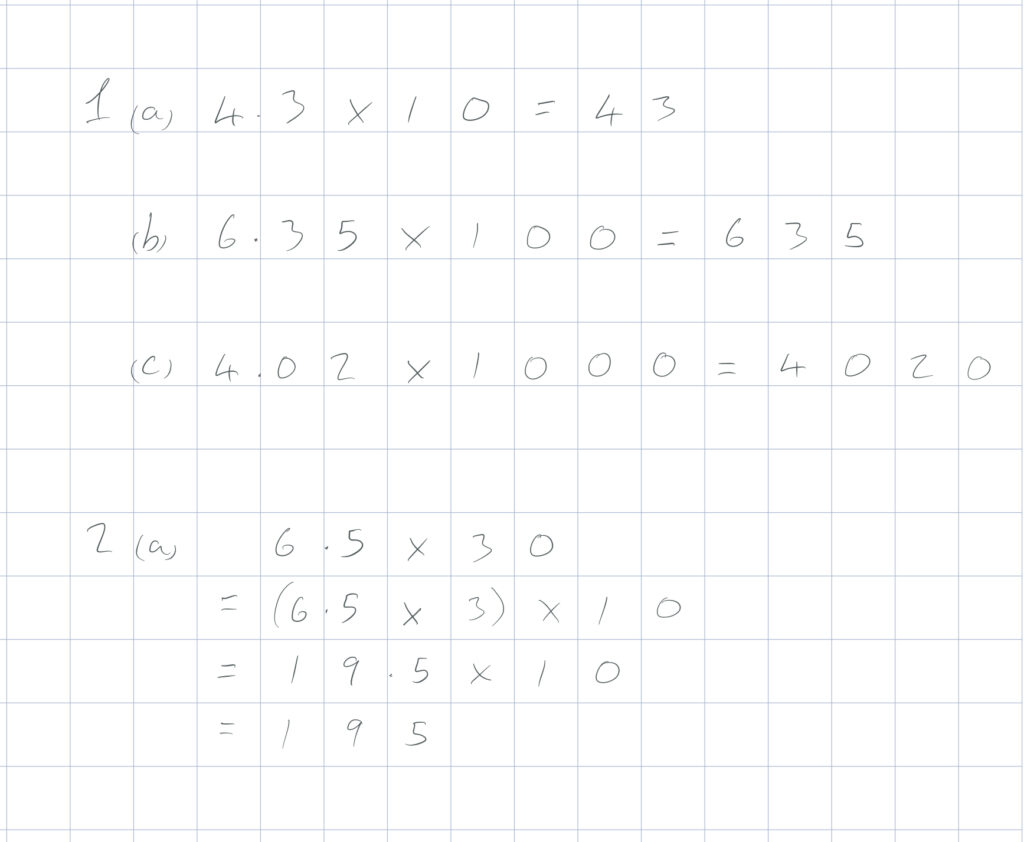To multiply numbers by 10, move the numbers one place to the left.

To multiply numbers by 100, move the numbers two places to the left.

To multiply numbers by 1000, move the numbers three places to the left.

To multiply numbers by multiples of 10, 100 or 1000, use two step multiplication (see video from 1:20).

Remember: always keep the digits in the same order.